# Starry Night Prom

How did I stand out at prom? In a light up dress of course!

IntermediateShowcase (no instructions)20,727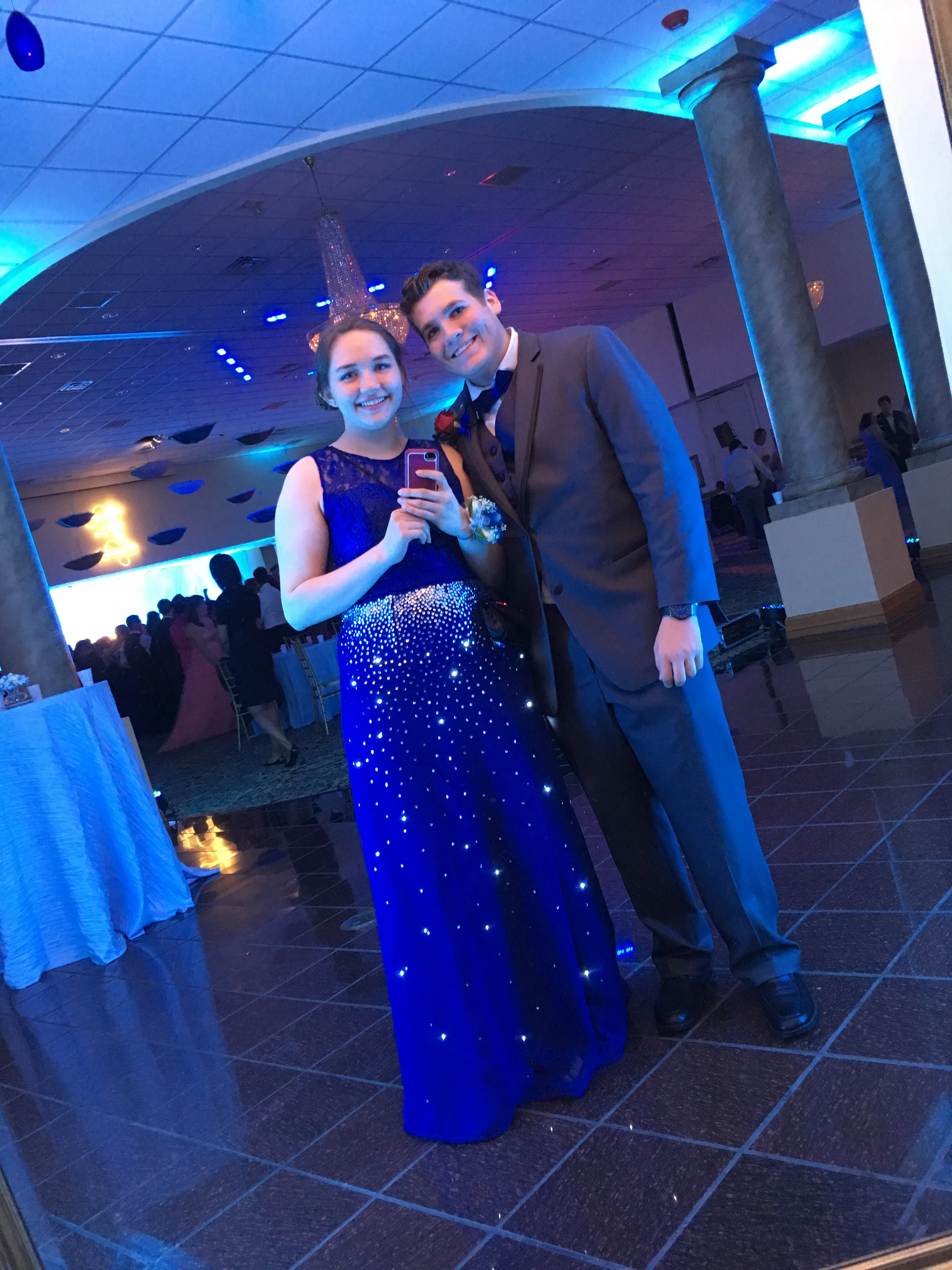## Things used in this project

### Hardware components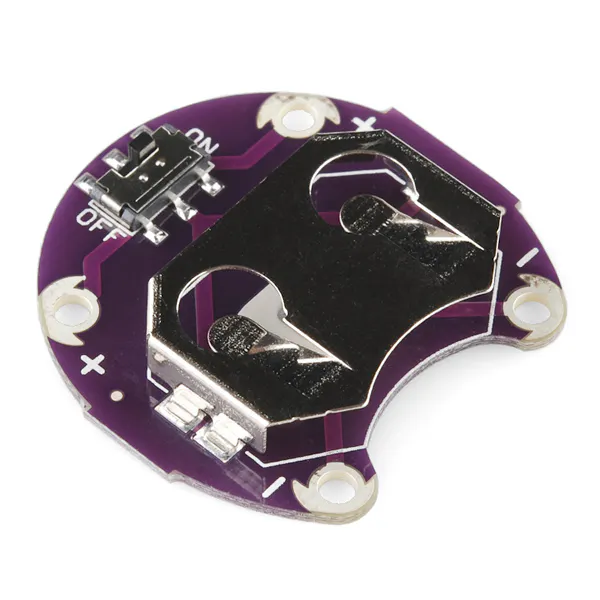SparkFun LilyPad Coin Cell Battery Holder - Switched - 20mm
×1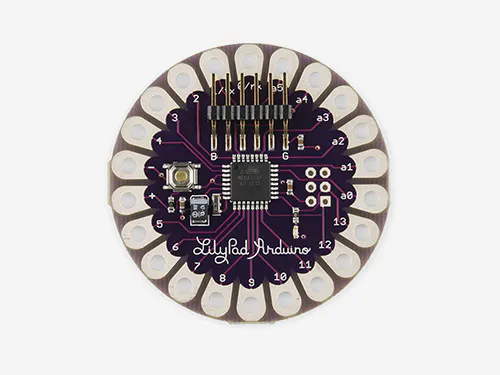×1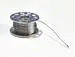×2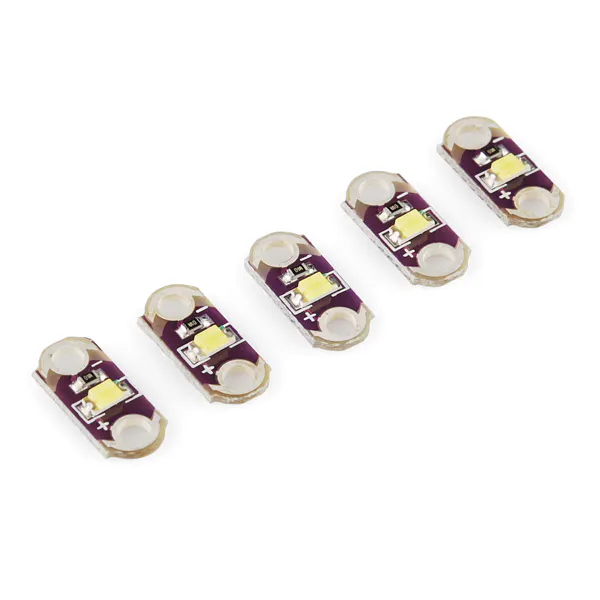×6Jumper wires (generic)
×1

## Schematics

### Dress Schematic

The actual layout of the wiring was a little more complicated, as there were really 30 LEDs hooked up the LilyPad, but they were in groups of varying size, each associated with one of the five shown LEDs. All the LEDs shared a ground and are controlled in the five groups by the signal wires connected to power of the LEDS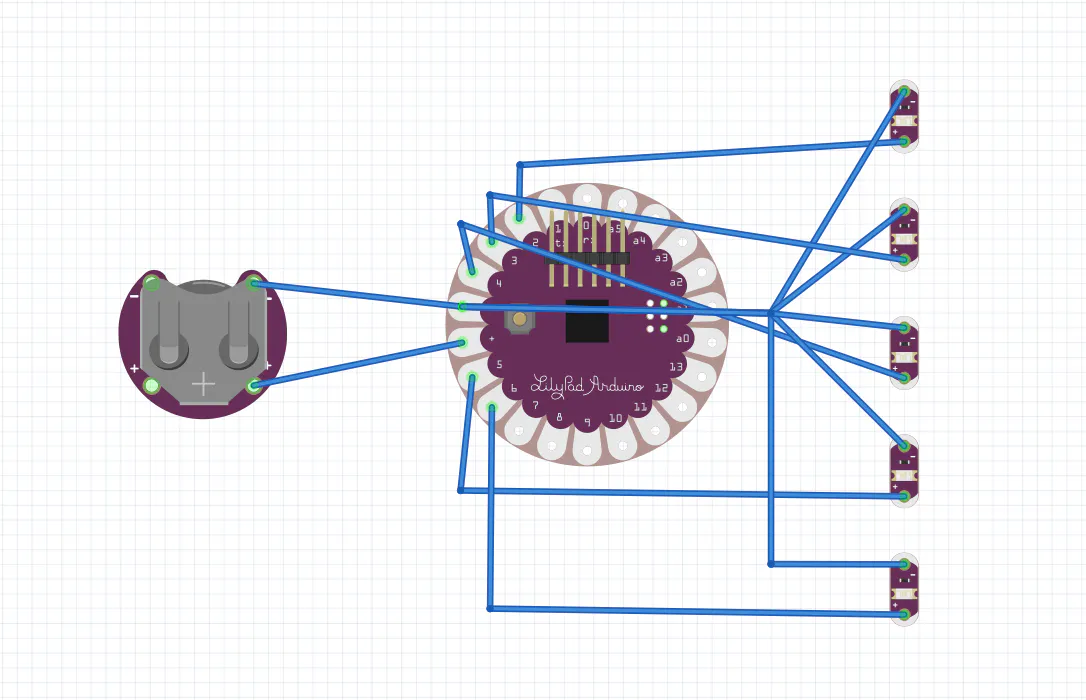## Code

### Dress Code

Arduino
Cycles through modes for making the lights do cool things
```//Maddy McGee
int list [] = {2, 3, 4, 5, 6};//ports used for lights
int cycle;
int cnter;//number of times a specific mode has ran
void setup() {
for (int cnt = 0; cnt < 5; cnt ++)
{
pinMode(list[cnt], OUTPUT);
}
cycle = 0;
cnter = 0;
}

void loop() {
if(cycle == 0)//decending lights
{
for (int cnt = 0; cnt < 5; cnt ++)
{
digitalWrite(list[cnt], HIGH);
delay(500);
digitalWrite(list[cnt], LOW);
}
cnter++;
if (cnter > 2)
{
cycle = 1;
cnter = 0;
}
}

if(cycle == 1)//decending lights, fast
{
for (int cnt = 0; cnt < 5; cnt ++)
{
digitalWrite(list[cnt], HIGH);
delay(100);
digitalWrite(list[cnt], LOW);
}
cnter++;
if (cnter > 15)
{
cycle = 2;
cnter = 0;
}
}

if(cycle == 2)//decending lights all ON, then all OFF
{
for (int cnt = 0; cnt < 5; cnt ++)
{
digitalWrite(list[cnt], HIGH);
delay(500);
}
delay(1500);
for (int cnt = 4; cnt >=0; cnt --)
{
digitalWrite(list[cnt], LOW);
delay(500);
}
cycle = 3;
}

if(cycle == 3)//acending lights
{
for (int cnt = 4; cnt >=0; cnt --)
{
digitalWrite(list[cnt], HIGH);
delay(500);
digitalWrite(list[cnt], LOW);
}
cnter++;
if (cnter > 2)
{
cycle = 4;
cnter = 0;
}
}

if(cycle == 4)//acending lights, fast
{
for (int cnt = 4; cnt >=0; cnt --)
{
digitalWrite(list[cnt], HIGH);
delay(100);
digitalWrite(list[cnt], LOW);
}
cnter++;
if (cnter > 15)
{
cycle = 5;
cnter = 0;
}
}

if(cycle == 5)//acending lights all ON, then all OFF
{
for (int cnt = 4; cnt >=0; cnt --)
{
digitalWrite(list[cnt], HIGH);
delay(500);
}
delay(1500);
for (int cnt = 0; cnt < 5; cnt ++)
{
digitalWrite(list[cnt], LOW);
delay(500);
}
cycle = 0;
}

}
```